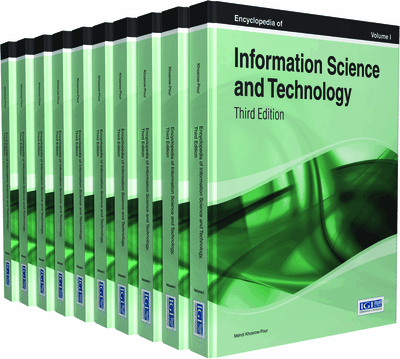# Expert Knowledge in Data Mining

Anthony Scime (The College at Brockport, State University of New York, USA)
DOI: 10.4018/978-1-4666-5888-2.ch171
Available
\$29.50
No Current Special Offers

Top

## Background

Data mining (also known as Knowledge Discovery from Data or KDD) is a term used to describe a number of analytical techniques that can be used to identify meaningful relationships in data (Fayyad, Piatetsky-Shapiro, & Smyth, 1996). Data mining models can make predictions for individual records using complex sets of rules found in the data. Additionally, data mining defines relationships in the data (Scime, Murray, Huang, & Brownstein-Evans, 2008; Chang, 2006). “In contrast to more conventional multivariate statistical methods such as factor analysis, principal component analysis, and multidimensional scaling, they [data mining techniques] tend to be less bound by a priori assumptions” (Spielman & Thill, 2008, p. 111).

Data mining is a data-intense analytical technique that is designed to exploit large data sets. It involves the analysis of data to find interesting patterns, confirm and probe previously known relationships, and detect previously unknown relationships in the data. Data mining models not only predict the results of a future event, but they also can provide knowledge about the structure and interrelationships among the data. It is these interrelationships that can lead to a better understanding of the data. As a discipline, data mining has its origins in artificial intelligence, machine learning, and statistics.

There are many data mining techniques. Three of the major techniques are classification, association, and clustering. Classification analysis constructs a decision tree model, finding a path to a predetermined dependent or target variable for each data record. A classification decision tree contains branches that can be converted to rules unique to the dataset, but applicable to future similar datasets. Research in data classification evolved from two sources. In statistics, CHAID (Chi-Squared Automatic Interaction Detection) (Kass, 1980) is a well known classification method that uses the chi-squared statistic to determine model structure. Machine learning research produced a number of classification methods, the best known of which is the C4.5 algorithm (Quinlan, 1993), which uses information gain to define the model’s structure. Both of these techniques produce a classification decision tree from which rules can be easily derived.

Association mining, which is a product of machine learning research, is used to find patterns of data that show conditions where sets of variables and their values occur frequently in the data set. With association mining, there is no predetermination of a target variable. Apriori (Agrawal, Imieliński, & Swami, 1993) is the predominant association mining algorithm. It is an algorithm that produces many rules, and domain expertise and special techniques are needed to reduce the rule set to those that are interesting and actionable.

Clustering is used to find groupings of data that show where data records occur in the multidimensional problem space, where each variable is represented as a dimension. It is often used to determine relationships between the data records. The most popular clustering algorithm is k-means (MacQueen, 1967). Again, analysis of the clusters needs special techniques and domain expertise.

## Key Terms in this Chapter

Data Dimensionality Reduction: The act of selecting attributes and instances to simplify the data without reducing the classification capabilities of the resultant model.

Record: A set of attributes that together define a single, unique entry in the data. Also known as a instance, entity, row, case, transaction, etc.

Classification Mining: A data mining method that constructs a model of the data’s behavior used to determine the expected classification of future instances. The model constructed from the data is a decision tree. The decision tree consists of decision nodes and leaf nodes, beginning with a root decision node, connected by edges. Each decision node is an attribute of the data and the edges represent the attribute values. The leaf nodes represent the dependent variable; the expected classification results of each data instance.

Information Gain: The change in information entropy from the current state of the set of instances to the proposed state of the set of instances. The entropy, H(s) , is a measure of the randomness of the distribution of the instances in a subset (s) of instances with respect to the dependent variable, d . where H(s) is the entropy of a set, s , and P(v i ) is the probability that v i is a value of attribute i .

Domain Expert: A person with a strong theoretical foundation in the specific field for which the data was collected. They understand the practical implications of the data, and can interpret the effect on the domain from the rules resulting from the data mining.

Association Mining: A data mining method that discovers frequent patterns, associations, correlations, or causal structures among sets of attributes in data sets. A frequent pattern is a pattern (set of attributes or a sequence) that occurs with some pre-established frequently in the data set.

Data Mining Life Cycle: This is a process involving human as well as computer resources in the conduct of a data mining project. It consists of 3 stages: hypotheses/objectives determination, data preparation, and data mining.

Attribute: A characteristic of an instance in the data. Also known as data element, field, item, data field, data item, column, etc.

## Complete Chapter List

Search this Book:
Reset